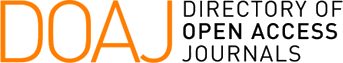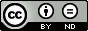### A Contribution to Triangulation Algorithms for Simple Polygons

Marko Lamot, Borut Žalik

#### Abstract

Decomposing simple polygon into simpler components is one of the basic tasks in computational geometry and its applications. The most important simple polygon decomposition is triangulation. The known algorithms for polygon triangulation can be classified into three groups: algorithms based on diagonal inserting, algorithms based on Delaunay triangulation, and the algorithms using Steiner points. The paper briefly explains the most popular algorithms from each group and summarizes the common features of the groups. After that four algorithms based on diagonals insertion are tested: a recursive diagonal inserting algorithm, an ear cutting algorithm, Kong’s Graham scan algorithm, and Seidel’s randomized incremental algorithm. An analysis concerning speed, the quality of the output triangles and the ability to handle holes is done at the end.

#### Full Text:

PDF

DOI: https://doi.org/10.2498/cit.2000.04.07